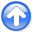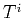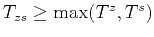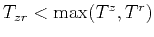First-break traveltime tomography with the double-square-root eikonal equationNext: Synthetic Model Examples Up: Li et al.: DSR Previous: DSR tomography

# Numerical Implementation

Following the analysis in Appendix A, we consider an implicit Eulerian discretization. For forward modeling, we solve the DSR eikonal equation by a version of the fast-marching method (FMM) (Sethian, 1999). First, a plane-wave withat subsurface zero-offsetis initialized. Next, in the update stage the traveltime at a grid point is computed from its upwind neighbors. A priority queue keeps track of the first-break wave-front, and the computation is non-recursive.

To properly handle the DSR singularity, we design an ordering of the combination of upwind neighbors during the update stage. Assuming thatis the upwind neighbor ofin the's direction for, we summarize the ordering as follows:

1. First try a three-sided update:
Solve equation A-9, returnif;
2. Next try a two-sided update: solve equations A-10, A-12 and A-13 and keep the results as,and, respectively.
Ifand, return;
Ifand, return;
Ifand, return;
3. Finally try a one-sided update:
Solve equation A-14, return.
An optional search routine A-17 may be added after the update to recover all branches of the DSR eikonal equation. The overall cost can be reduced roughly by half by acknowledging the source-receiver reciprocity and thus computing only the positive (or negative) subsurface offset region.

For an implementation of linearized tomographic operators 12 and 16, we choose upwind approximations (Franklin and Harris, 2001; Li et al., 2011; Lelièvre et al., 2011) for the difference operators in equation 8. In Appendix C, we show that the upwind finite-differences result in triangularization of matrices 11 and 15. Therefore, the costs of applyingandand their transposes are inexpensive. Moreover, although our implementation belongs to the family of adjoint-state tomographies, we do not need to compute the adjoint-state variable as an intermediate product for the gradient.

Additionally, the Gauss-Newton approach approximates the Hessian in equation 6 by. An updateat currentis found by taking derivative of equation 6 with respect to, which results in the following normal equation:(17)

To add model constraints, we combine equation 17 with Tikhonov regularization (Tikhonov, 1963) with the gradient operator and use the method of conjugate gradients (Hestenes and Stiefel, 1952) to solve for the model update.First-break traveltime tomography with the double-square-root eikonal equationNext: Synthetic Model Examples Up: Li et al.: DSR Previous: DSR tomography

2013-10-16